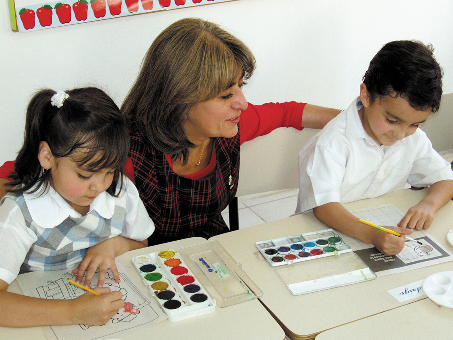,
Message sent from:# Primary – Numeracy

Key stage 1 curriculum

 Autumn Term 1 Autumn Term 2 Spring Term 3 Spring Term 4 Summer Term 5 Summer Term 6 Numeracy Year 1 Number – Number and Place value Number – Addition and Subtraction Measure and Geometry –  Shapes and Patterns, Length and Height Number – Divisions and fractions Number – Place value, addition and subtraction Multiplicat-ion and measure-ment – Multiplicat-ion, time, volume and capacity Year 2 Number – Number and place value, addition and subtraction Number – Multiplicat-ion and division Measure – Mass, Length and Temperature Geometry and number – 2D shapes and fractions Number and measure – Time and volume Measure – Position and direction

Key Stage 2 curriculum

 Autumn Term 1 Autumn Term 2 Spring Term 3 Spring Term 4 Summer Term 5 Summer Term 6 Numeracy Year 3 Number – Number and place value, addition and subtraction Number – Multiplicat-ion and division Measure and geometry – Length and time Number – Fractions and statistics Measurement – Volume and capacity Geometry – Perimeters, angles, lines and shapes Year 4 Number – Number and place value, addition and subtraction Number – Multiplicat-ion and division Measure and geometry – Mass, volume and length, geometry Number – Fractions, decimals and statistics Measurement – Time Measure and geometry – Area of figures, position and movement Year 5 Number – Number and place value, addition and subtraction Number – Multiplicat-ion and division Number – Fractions and decimals Geometry – Properties of shapes; position and direction Measurement – Measurements, area and perimeter, volume Number and statistics – Percenta-ge and graphs Year 6 Number – Number and place value, the four operations Number – Fractions, including decimals and percenta-ges Number and measure-ments – Ratio and proportions, measure-ments Geometry, measurement and number – Area and perimeter, volume, algebra Geometry and Number – Position and movement, word problems Statistics and Number – Graphs and averages, negative numbers

X